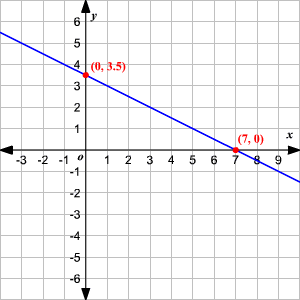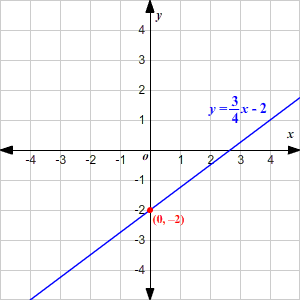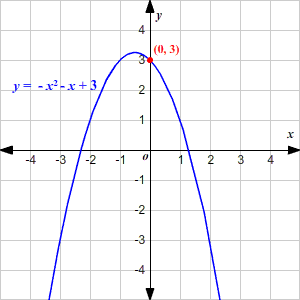# $y$ -Intercepts

The $y$ -intercept of a graph is the point where the graph crosses the $y$ -axis. (Because a function must pass the vertical line test , a function can have at most one $y$ -intercept . )

The $y$ -intercept is often referred to with just the $y$ -value. For example, we say that the $y$ -intercept of the line shown in the graph below is $3.5$ .When the equation of a line is written in slope-intercept form ( $y=mx+b$ ), the $y$ -intercept $b$ can be read immediately from the equation.

Example 1:

The graph of $y=\frac{3}{4}x-2$ has its $y$ -intercept at $-2$ .Similarly, for a quadratic equation written in standard form $y=a{x}^{2}+bx+c$ , the $y$ -intercept is $c$ .

Example 2:

The graph of $y=-{x}^{2}-x+3$ has its $y$ -intercept at $3$ .